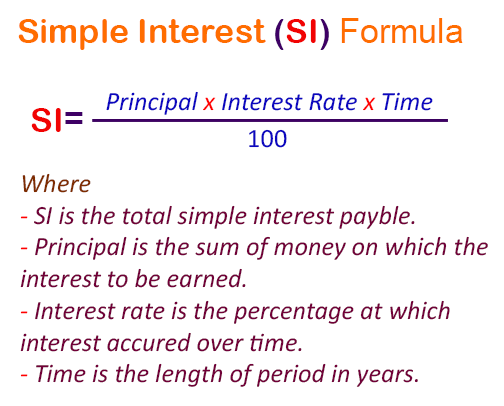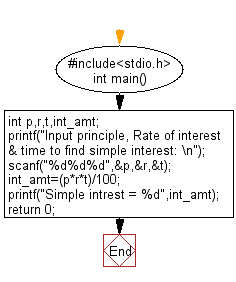﻿ C Program: Compute the simple interest - w3resource

# C Exercises: Compute the simple interest

## C Basic Declarations and Expressions: Exercise-53 with Solution

Write a C program that accepts the principle, rate of interest, and time and calculates simple interest.

Pictorial Presentation:Sample Solution:

C Code:

``````#include<stdio.h>
int main() {
int p, r, t, int_amt;

// Prompt user to input principle, rate of interest, and time
printf("Input principle, Rate of interest & time to find simple interest: \n");
scanf("%d%d%d", &p, &r, &t);

// Calculate simple interest
int_amt = (p * r * t) / 100;

// Display the calculated simple interest
printf("Simple interest = %d", int_amt);

return 0;
}
``````

Sample Output:

```Input Data: p = 10000, r = 10% , t = 12 year
Input principle, Rate of interest & time to find simple interest:
Simple interest = 12000
```

Flowchart:C programming Code Editor:

What is the difficulty level of this exercise?

Test your Programming skills with w3resource's quiz.

﻿